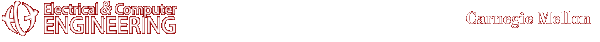#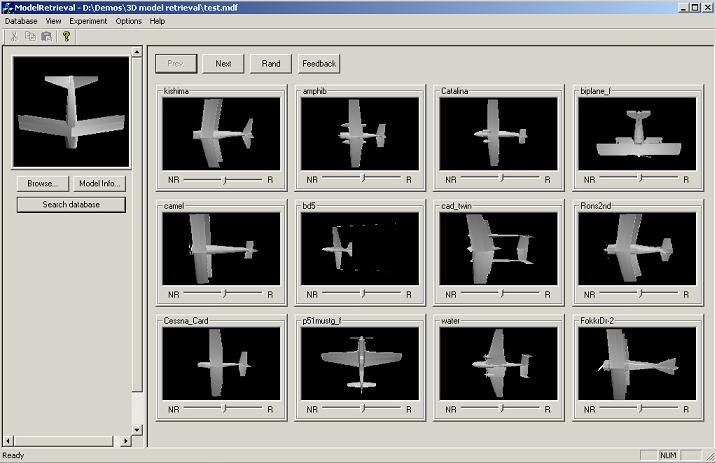Cha Zhang czhang@andrew.cmu.edu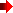Top of this page

3D scene/object browsing is becoming more and more popular as it engages people with much richer experiences than 2D images. As it is easy to build 3D models from real scene/object today, more and more 3D models are available over the Internet. The growing size of available 3D models makes 3D model retrieval very important. This work focuses on extracting features that well represent 3D models and combining these features in order to get a better retrieval performance.

Work has been done to retrieve similar 3D models from a database. As many other content-based information retrieval systems, a 3D model retrieval system extracts several low-level features for each model, and measures the similarity between any two models in the low-level feature space. Cord histogram, shape index and some rotation invariant shape descriptors are the commonly used features. In our system, we propose a new set of features that view the 3D model as a solid binary region. Ten features such as volume-surface ratio, aspect ratio, moment invariants and Fourier transform coefficients are extracted. In the current version, we simply use Euclidean distance to measure the similarity between two models after the features are normalized .Top of this page

3D model data collection

The 3D models we have in our database are mainly collected from the MPEG-7 3D shape core experiments, the RcCad company (http://www.rccad.com), the VRML object supermarket in UK (http://www.dcs.ed.ac.uk/home/objects/vrml.htmll) and the Rother Daten Service in Germany (http://www.rdservice.de/german/produkte/VRCreator/library/vrlibrary.html). The total number of models is around 2,000. All the 3D models are in Virtual Reality Modeling Language (VRML) format, which uses mesh models to represent the 3D content. We use VRML because it is rapidly becoming the standard file format for the delivery of 3D contents across the Internet.

Feature extraction

We propose a new set of features that view the 3D model as a solid binary region. Compared with the other features, region-based e propose to calculate region features from the mesh representation directly. We calculate a feature for a model by first finding it for the elementary shapes, such as triangles or tetrahedrons, and then add them up. The computational complexity is proportional to the number of elementary shapes, which is typically much smaller than the number of voxels in the equivalent volumetric representation. Both 2D and 3D meshes are considered. The result is general and has many potential applications.

To calculate region-based features, the 3D model has to be closed. Otherwise, surface close algorithm has to be performed first. Fortunately, it is easy to close a 3D mesh model. After the triangulation, a typical edge is associated with even number of triangles, while for a boundary edge, the number of triangles associated with it is odd.  We close the 3D model by connecting boundary edges, which is shown in the following figure: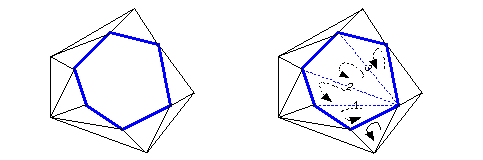Notice that when we record the order of the vertices of a newly added triangle, we need to pay attention to make the normals of the triangles consistent.

We calculate the moments and Fourier transforms of triangles and tetrahedrons. Here are the up to 3rd order moments for triangles and tetrahedrons:

2D case - (0, 0), (x1, y1), (x2, y2) are the three vertexes of the triangle.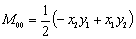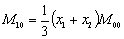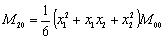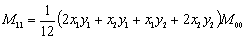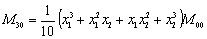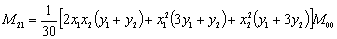3D case - (0, 0, 0), (x1, y1, z1), (x2, y2, z2), (x3, y3, z3) are the four vertexes of the tetrahedron.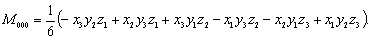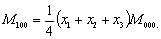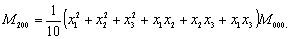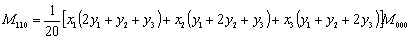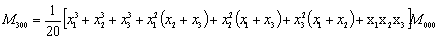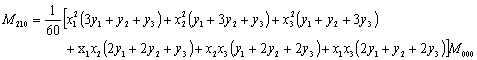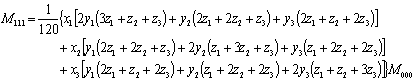Here are the Fourier transforms of triangles and tetrahedrons:

2D case - (0, 0), (x1, y1), (x2, y2) are the three vertexes of the triangle.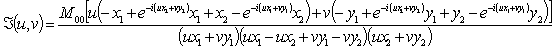3D case - (0, 0, 0), (x1, y1, z1), (x2, y2, z2), (x3, y3, z3) are the four vertexes of the tetrahedron.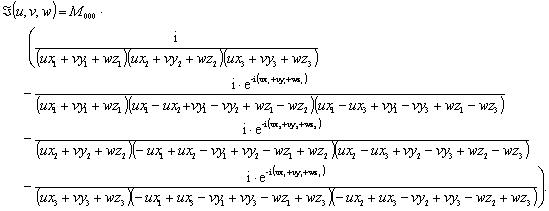Similarity measurement

In the current system, we simply normalize the features and use Euclidean to measure the similarity. Better algorithms will be plugged in later.Top of this page

• We are sorry, the demo of this project is not available yet.Top of this pageTop of this page

Any suggestions or comments are welcome. Please send them to Cha ZhangTop of this page